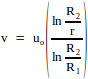# A long cylinder of radius Ris displaced along its axis with a constant velocity u0 inside a stationary coaxialcylinder of radius R2. The space between the cylinders is filled with a viscous liquid. Find the velocity of the liquid as a function of the distance r from the axis of the cylinders.The flow is laminar.

7 years ago
p { margin-bottom: 0.25cm; line-height: 120%; }

Since the flow is laminar, the viscous force acting between the liquid layers is given by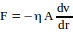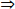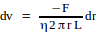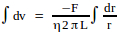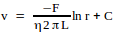substituting v = 0 when r = R2, we get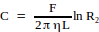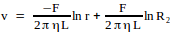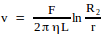eq (1)

We also know that v = uo when r = R1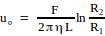eq (2)

dividing eq(1) by eq(2), we get